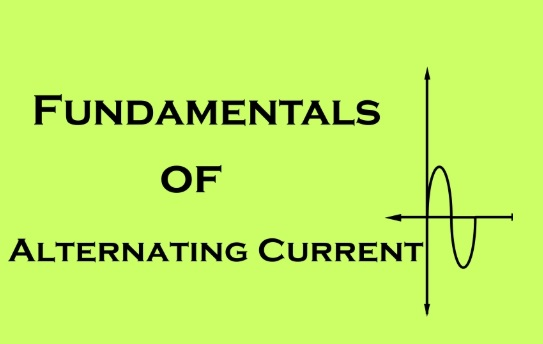# FUNDAMENTALS OF ALTERNATING CURRENTSWe have dealt so far with cases in which the currents are steady and is in one direction; this is called direct current ( d.c.) the use of direct currents is limited to a few applications. e.g. charging of batteries, electroplating, electric
traction etc. For large scale power distribution there are however many advantages in using alternating current (a.c.) In an a.c. system, the voltage acting in the circuit changes polarity at regular intervals of time and the resulting current (called alternating current) changes direction accordingly. The ALTERNATING CURRENTS system has offered so many advantages that at present electrical energy is universally generated, transmitted and used in the form of alternating current. Even when d.c. energy is necessary, it is common practice to convert A.C. into D.C. by means of rotary converters or rectifiers

### Alternating Current :

The alternating current is that current which flows in a conductor in one direction for some time and immediately it flows, in the opposite direction for sometime. The shape of the wave form obtained decides the type of alternating quantity or current or voltage.

### Production of Alternating E.M.F. :

Alternating voltage may be produced by rotating a coil in a magnetic field as shown in figure.## Fundamentals of Alternating Currents

N, S = Magnets P,Q, R,S = Rectangular
AB = Load a,b = carbon brushes

The Value of the voltage produced depends upon the number of turns of the coil, strength of the field and the speed at which the coil rotates. The emf induced depends upon the Faraday laws of electro magnetic Induction. which
state that whenever a conductors cuts across a magnetic lines of force or flux an emf is induced in it. Also the magnitude of the induced emf is directly proportional to the rate of change of flux linkages.### Different wave forms :

The shape or curve obtained by plotting the instantaneous values of voltage or current is called its wave forms. The different wave forms are as shown in the fig### Cycle :

One complete set of positive and negative values of an alternating quantity is called a cycle OABCD is a cycle in fig### Periodic Time :

The time taken by the wave to complete a cycle is called periodic Time it is denotted by ‘T’ If 50 cycle are obtained in one second then the time period or periodic time for one cycle is 1/ 50 second or 0.02 seconds.### Frequency :

The number of cycles per second is called frequency or periodicity.
Time in secondsThe unit of frequency is hertz and the symbol used is Hz. It is reciprocal of the time period### Instantaneous value :

The magnitude at a given time is called the instantaneous value. For example at time op the instantaneous value is PR. It varies from instant to instant on the wave. Small letters ( i and v ) are used to indicate instantaneous value of
current and voltage. or The value at any particular instant is called instantaneous value.### Average Value :

It is the steady state current ( D.C. ) which transfer across any circuit the same charge as it is transferred by that alternating quantity ( A.C.) the average value Iav over the complete cycle is zero. Therefore the average value is
obtained for half cycle only or the average of the instant value in one half of the cycle is known as the average value### R.M.S. Value :

The RMS value of alternating current is given that steady d.c. current which produces same heat as that produced by the alternating current passing through on given resistance for the same period of time.

Ir. m.s = 0.707 Imax

### Maximum Value :

This is also called crest value, peak value or amplitude. It is the highest value attained by the current or voltage in a half cycle### Form Factor :

Form factor of an A.C. is the ratio between R.M.S. value and mean value or Average value.### Peak Factor :

It is the ratio of maximum, value to R.M.S. Value### Phasor Representation :

Electrical quantities like voltage and current are represented by means of vectors with the length representing the magnitude and the arrow representing the direction. The vectors are assumed to rotate in anti – clock wise direction.### Phase :

The angular displacement between two or more alternating quantities is known as phase or The meaning of phase is to indicate the relative position between voltage and current components. The components may rise or fall at the same time may lag the other or lead.